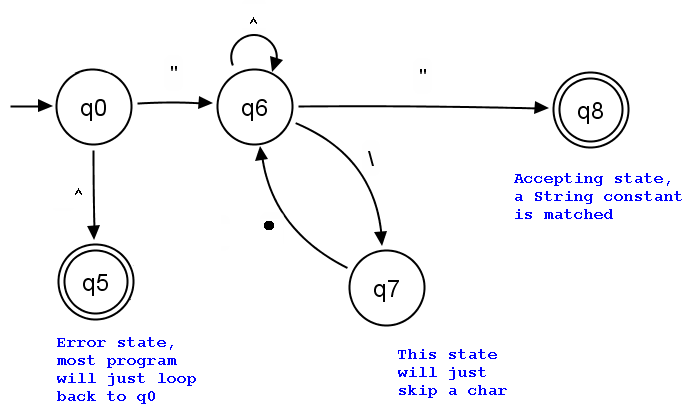## Sunday, June 9, 2013

### Playing a Wave file

This is in response to a reader's comment in Sound Programming - Part 2.

I am going to show how to play a sound file using similar API as Beep.java and FadeBeep.java

This is part 5 of the series. If you are interested in the whole series, go to the Featured page.

### A wave file player with Fading out Effect

Since the comment is under FadeBeep.java, I would assume the reader wants a fading out effect while playing the sound file. Here is a sample that works for 16-bit wave file.

## Saturday, June 8, 2013

### Introduction

In Java Comment Highlighter, I have demonstrated how to highlight all Java comment in a text file, using the regular expression technique.

I am now going to use similar technique to write a String highlighter in Java, which will highlight all quoted string in a Java source file. The program will read a text file (a.txt) containing Java source code and convert it to a.html, with all String literals highlighted. We will use HtmlWriter.java as a starting point. You are recommended to read it first.

### Replacement with Regular Expression

The regular expression of matching a quoted String in Java is :

It is not very obvious. I will try to explain it below.

### Explaining the Regular Expression

Regular ExpressionExplanation
``` "```
Match a quote first
``` [^"\\]*```
then repeatedly skipping characters until a quote or a stroke is read
``` (```
if we read a stroke, then begin
``` \\.```
skip one character following the stroke
``` [^"\\]*```
once again, repeatedly skipping characters until a quote or a stroke is read
``` )```
end if a storke is read
``` *```
Repeat the above bracket group until no more stroke is found before the ending quote
``` "```
Finally, match the ending quote
The regular expression is actually :
```"[^"\\]*(\\.[^"\\]*)*"
```
Optionally, to optimize it a little bit, we may add a question mark and a colon to mark a non-capturing group.
```"[^"\\]*(?:\\.[^"\\]*)*"
```

To store the regular expression in a Java String, we need to escape all the quote and stroke characters. Hence we have :

### Deriving the regular expression from DFA

This is another way of deriving the regular expression of a quoted String. We will derive it by drawing a state diagram of a imaginary automatic machine that will match the quoted String. DFA stands for Deterministic Finite Automata. It is Finite because it has finite number of states. It is deterministic because for every input character it know where to go. DFA has a strict definition which is easy to be found on the web. Hence I will just draw the state diagram here.### State Transition

If we are in q0 and read a quote, we will go to q6
We represent the above transition by:
```  q0" => q6
```
If we are in q6 and read a quote, we will go to q8
```  q6" => q8
```
If we are in q6 and read a stroke, we will go to q7
```  q6\ => q7
```
f we are in q6 and read a char other than a quote or a stroke, then we will go back to q6
```  q6[^"\] => q6
```
If we are in q7 and read any char, we will go to q6
```  q7. => q6
```
The dot means any character in the alphabet. Let's define the alphabet to be the set of all ASCII characters excluding the new line character.

### Full list of Transitions

```(1)     q6" => q8
(2)     q0" => q6
(3)     q7. => q6
(4) q6[^"\] => q6
(5)     q6\ => q7
```
We may combine (2), (3) and (4) to form a new transition by using the union character.
```(6) q0"|q7.|q6[^"\] => q6
```
Looking at the third union, we are forming a loop. We will use the * character to represent the loop.
```(7) (q0"|q7.)[^"\]* => q6
```
Rewriting it by distributing the star to each union, we have:
```(8) q0"[^"\]*|q7.[^"\]* => q6
```
From (5), we may substitute q7 with `q6\`
```(9) q0"[^"\]*|q6\.[^"\]* => q6
```
Now we have another loop, so we use the star to represent the loop again.
```(10) q0"[^"\]*(\.[^"\]*)* => q6
```
We may now substitute (10) into (1)
```(1)  q6" => q8  that means :
(11) q0"[^"\]*(\.[^"\]*)*" => q8
```
That means starting from the initial state q0, we may arrive at the final state q8 by the regular expression:
```  "[^"\\]*(\\.[^"\\]*)*"
```
Note that stroke is a meta character in regular expression and hence I have escaped all the stroke characters (become double strokes)

The expression derived by the DFA is the same as the one I explained above.

### Highlighting the String

Once we have the regular expression ready, it is simple to implement a String highlighter. Since the implementation is very similar to the comment highlighter, I will not repeat here.

## Saturday, June 1, 2013

### Base64 Encoder and Decoder in Java

Introduction

There are many third party libraries providing Base64 encoding and decoding functions. Many of them are open source solution. I am not going to say too much on the topic. I would just provide my implementation here. If you want to know more, go to the wiki page.

Source

The source contains over 200 lines, however about half of them are comments and unit test. Hence the encode() and decode() function are not really complex. In case you find reading other programmer code difficult. I would provide a typical way of how I read other people code.

Read the comment header first, then scroll down to the main program to see how to use the functions. Then read the functions following the flow in main().